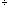I would like to know the full answer on how to turn the fraction 3 3/8 into a decimal please. Sincerely Hi, I think the easiest way is to convert the fraction 3/8 into decimal form by dividing 8 into 3. 38 = 0.375 and thus 3 3/8 = 3 + 0.375 = 3.375 Penny